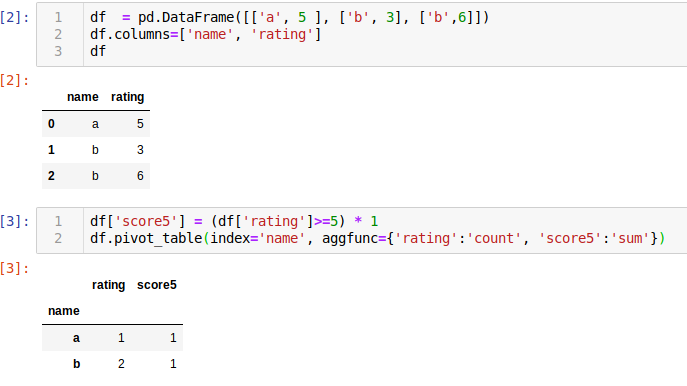# Summarize dataframe by extracting and grouping column with pandas

Hello,

I wonder if there is any method I could use to group column data…
I tried “df.groupby(‘website’).agg(‘rating’)”
but I don’t know how to implement the count, nor do I know how to print the dataframe

Here is my data,
idx Website_name rating
1 a 5
2 b 3
3 b 6

expected output:
website count val_rate(the number of rate >= 5)
a 1 1
b 2 1

probably not the cleanest way of doing it, you can probably pivot/group_by with lambdas but this works:for copying:

``````df['score5'] = (df['rating']>=5) * 1
df.pivot_table(index='name', aggfunc={'rating':'count', 'score5':'sum'})
``````
1 Like

Thank you! is this from panda library?

also – do you have any idea if I could get data with from_folder and from_df at the same time?